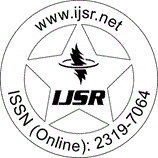International Journal of Science and Research (IJSR)
Call for Papers | Fully Refereed | Open Access | Double Blind Peer Reviewed

Research Paper | Mathematics | Kenya | Volume 10 Issue 11, November 2021

# Quasiaffine Inverses of Linear Operators in Hilbert Spaces

J. M. Mwanzia | M. Kavila | J. M. Khalagai

Abstract: Abstract: Let H denote a complex Hilbert space and B (H) denote the Banach algebra of bounded linear operators on H. Given operators A, B, X ∈ B (H), we define R (A, B) : B (H) → B (H) by R (A, B) X = AXB - X and C (A, B) : B (H) → B (H) by C (A, B) X = AX - XB. In this paper, we investigate properties of the operators A, B ∈ B (H) satisfying R (A, B) X = 0 or R (B, A) Y = 0 or both where X and Y are one-one or have a dense range or both. In particular, the case R (A, B) X = 0 = R (B, A) Y is of special interest with respect to invertibility of the operator A under some classes of operators.

Keywords: quasiaffinity, quasiaffine inverse and invertibility of operators

Edition: Volume 10 Issue 11, November 2021,

Pages: 1076 - 1082